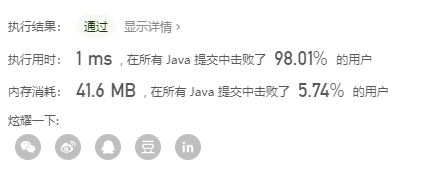# LeetCode#26-删除排序数组中的重复项

package shuangzhizhen;
/*
26. 删除排序数组中的重复项

*/
public class p26 {
public int removeDuplicates(int[] nums) {
int n=nums.length;
if(n==0)return 0;
if(n==1)return 1;
int index=1;
for(int left=1;left<n;left++){
if(nums[left]!=nums[left-1]){
nums[index++]=nums[left];
}

}
return index;

}

}


运行结果：posted @ 2020-07-13 18:11  菜鸡要加油  阅读(5)  评论(0编辑  收藏